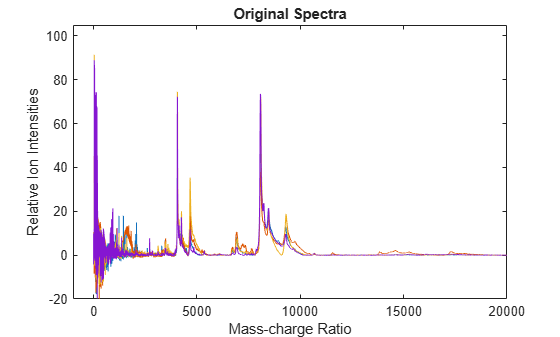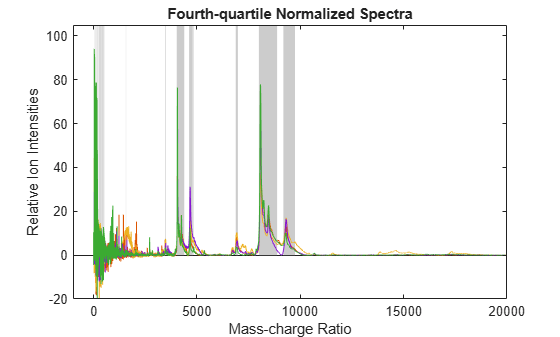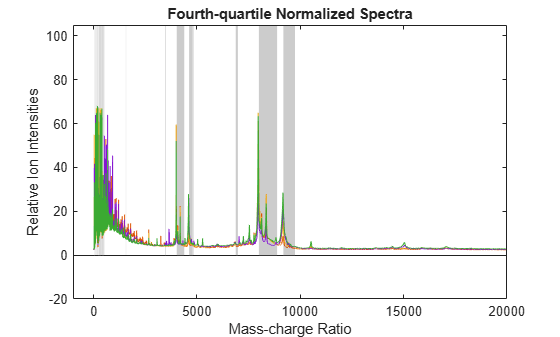# msnorm

Normalize set of signals with peaks

## Syntax

``yOut = msnorm(X,Intensities)``
``[yOut,normParams] = msnorm(X,Intensities)``
``yOut = msnorm(X,Intensities,NormParameters)``
``[___] = msnorm(X,Intensities,Name,Value)``

## Description

example

````yOut = msnorm(X,Intensities)` normalizes a group of signals with peaks by standardizing the area under the curve (AUC) to the group median and returns the normalized data `yOut`.```

example

````[yOut,normParams] = msnorm(X,Intensities)` also returns the normalization parameters `normParams`, which you can use to normalize another group of signals.```

example

````yOut = msnorm(X,Intensities,NormParameters)` uses the parameter information `NormParameters` from a previous normalization to normalize a new set of signals. The function uses the same parameters to select the separation-unit positions and output scale from the previous normalization. If you specified a consensus proportion using the `'Consensus'` name-value pair argument in the previous normalization, the function selects no new separation-unit positions and performs normalization using the same separation-unit positions.```

example

````[___] = msnorm(X,Intensities,Name,Value)` uses additional options specified by one or more name-value pair arguments and returns any of the output arguments in previous syntaxes. For example, ```out = msnorm(X,Y,'Quantile',[0.9 1])``` sets the lower (0.9) and upper (1) quantile limit to use only the largest 10% of intensities in each signal to compute the AUC.```

## Examples

collapse all

This example shows how to normalize the area under the curve of every mass spectrum from the mass spec data.

Load a MAT-file, included with the Bioinformatics Toolbox™ software, that contains sample mass spec data, including MZ_lo_res, a vector of m/z values, and Y_lo_res, a matrix of intensity values.

`load sample_lo_res`

Create a subset (four signals) of the data.

```MZ = MZ_lo_res; Y = Y_lo_res(:,[1 2 5 6]);```

Plot the four spectra.

```plot(MZ, Y) axis([-1000 20000 -20 105]) xlabel('Mass-charge Ratio') ylabel('Relative Ion Intensities') title('Original Spectra')```Normalize the area under the curve (AUC) of every spectrum to the median, eliminating low-mass (m/z < 1,000) noise, and post-rescaling such that the maximum intensity is 100. Plot the four spectra.

```Y1 = msnorm(MZ,Y,'Limits',[1000 inf],'Max',100); plot(MZ, Y1) axis([-1000 20000 -20 105]) xlabel('Mass-charge Ratio') ylabel('Relative Ion Intensities') title('AUC Normalized Spectra')```This example shows how to normalize the ion intensity of every spectrum from the mass spec data.

Load a MAT-file, included with the Bioinformatics Toolbox™ software, that contains sample mass spec data, including MZ_lo_res, a vector of m/z values, and Y_lo_res, a matrix of intensity values.

`load sample_lo_res`

Create a subset (four signals) of the data.

```MZ = MZ_lo_res; Y = Y_lo_res(:,[1 2 5 6]);```

Normalize the ion intensity of every spectrum to the maximum intensity of the single highest peak from any of the spectra in the range above 1000 m/z. Plot the four spectra.

```Y2 = msnorm(MZ,Y,'QUANTILE', [1 1],'LIMITS',[1000 inf]); plot(MZ, Y2) axis([-1000 20000 -20 105]) xlabel('Mass-charge Ratio') ylabel('Relative Ion Intensities') title('Maximum-Intensity Normalized Spectra')```This example shows how to perform quantile normalization for mass spec data.

Load a MAT-file, included with the Bioinformatics Toolbox™ software, that contains sample mass spec data, including MZ_lo_res, a vector of m/z values, and Y_lo_res, a matrix of intensity values.

`load sample_lo_res`

Create a subset (four signals) of the data.

```MZ = MZ_lo_res; Y = Y_lo_res(:,[1 2 5 6]);```

Normalize using the data in the m/z regions where the intensities are within the fourth quartile in at least 90% of the spectrograms. Note that you can use the normalization parameters in the second output to normalize another set of data in the same m/z regions. Plot the four spectra.

```[Y3,S] = msnorm(MZ,Y,'Quantile',[0.75 1],'Consensus',0.9); area(MZ,S.Xh.*1000,'LineStyle','None','FaceColor',[.8 .8 .8]) hold on plot(MZ, Y3) hold off axis([-1000 20000 -20 105]) xlabel('Mass-charge Ratio') ylabel('Relative Ion Intensities') title('Fourth-quartile Normalized Spectra')```Use the normalization parameters in the second output of the previous step to normalize a different subset of data (four signals) using the data in the same m/z regions as the previous data set. Plot the four spectra.

```Y4 = msnorm(MZ,Y_lo_res(:,[3 4 7 8]),S); area(MZ,S.Xh.*1000,'LineStyle','None','FaceColor',[.8 .8 .8]) hold on plot(MZ, Y4) hold off axis([-1000 20000 -20 105]) xlabel('Mass-charge Ratio') ylabel('Relative Ion Intensities') title('Fourth-quartile Normalized Spectra')```## Input Arguments

collapse all

Vector of separation-unit values for a set of signals with peaks, specified as a vector.

Data Types: `double`

Intensity values for a set of peaks that share the same separation-unit range, specified as a matrix. Each row is a separation-unit value and each column is either a set of signals with peaks or a retention time. The number of rows in `Intensities` must equal the number of elements in the input vector `X`.

Data Types: `double`

Normalization parameters to normalize another group of signals, specified as a structure. `NormParameters` is a structure returned by `msnorm` from a previous normalization call.

### Name-Value Arguments

Specify optional pairs of arguments as `Name1=Value1,...,NameN=ValueN`, where `Name` is the argument name and `Value` is the corresponding value. Name-value arguments must appear after other arguments, but the order of the pairs does not matter.

Before R2021a, use commas to separate each name and value, and enclose `Name` in quotes.

Example: `out = msnorm(X,Y,'Quantile',[0.9 1])` sets the lower (0.9) and upper (1) quantile limit to use only the largest 10% of intensities in each signal to compute the AUC.

Quantile limits to reduce the set of separation-unit values in `X`, specified as a `1`-by-`2` vector or a scalar between `0` and `1`.

If you specify a vector, the first element is the lower limit and the second element is the upper limit. For example, `[0.9 1]` means that the function uses only the largest 10% of intensities in each signal to compute the AUC. The default value `[0 1]` means that the function uses the whole AUC, instead of limiting the intensities to a particular quantile.

If you specify a scalar value, it represents the lower quantile limit. The upper quantile limit is automatically set to `1`.

Example: `'Quantile',[0.8 1]`

Data Types: `double`

Separation-unit range to pick normalization points, specified as a `1`-by-`2` vector. The default value `[min(X) max(X)]` selects all available points from `X`. If you specify a lower or upper limit as a value that is not within the available range `[min(X) max(X)]`, the function sets the lower limit to `min(X)` and the upper limit to `max(X)`.

This parameter is useful to eliminate noise from the AUC calculation. For instance, you can exclude the matrix noise that appears in the low-mass region (m/z values less than `1000`) of a SELDI mass spectrometer by setting the limit to `[1000 max(X)]`.

Example: `'Limits',[900 max(X)]`

Data Types: `double`

Minimal percentage of intensity values within the quantile limits that a separation-unit position must have to be included in the AUC calculation, specified as a scalar between `0` and `1`. The same separation-unit positions are then used to normalize all the signals. Use this parameter to eliminate low-intensity peaks and noise from the normalization.

For instance, to select m/z regions whose intensities are within the third quantile in at least 90% of the spectrograms, set `'Quantile'` and `'Consensus'` as follows: ```yOut = msnorm(MZ,Y,'Quantile',[0.5 0.75],'Consensus',0.9)```.

Example: `'Consensus',0.8`

Data Types: `double`

Method for normalizing the AUC of every signal, specified as `'Median'` or `'Mean'`.

Example: `'Method','Mean'`

Data Types: `char` | `string`

Overall maximum intensity to scale to after normalizing each signal individually, specified as a scalar. If you do not specify this parameter, no postscaling is performed.

Note

If you specify this value and also set `'Quantile'` to `[1 1]`, then a single point (peak height of the tallest peak) is normalized to the specified maximum value.

Example: `'Max'`

Data Types: `double`

## Output Arguments

collapse all

Normalized intensity values, returned as a matrix.

Normalization parameters that you can use to normalize another group of signals, returned as a structure.

## Version History

Introduced before R2006a